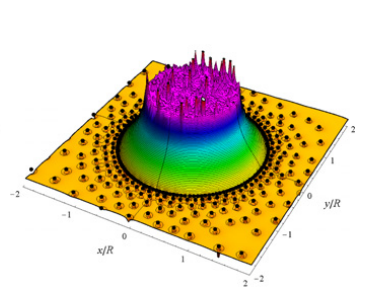## Monte Carlo simulations of two-component Coulomb gases applied in surface electrodes

#### J. Phys.: Condens. Matter 34 144007 (2022)

In this work, we study the gapped surface electrode (SE), a planar system composed of two-conductor flat regions at different potentials with a gap between both sheets. The computation of the electric field and the surface charge density requires solving Laplace’s equation subjected to Dirichlet conditions (on the electrodes) and Neumann boundary conditions over the gap. In this document, the gapless surface electrode is modeled as a two-dimensional classical Coulomb gas having punctual charges +q and −q on the inner and outer electrodes, respectively, interacting with an inverse power law 1/r-potential. The coupling parameter Γ between particles inversely depends on temperature and is proportional to q2. Precisely, the density charge arises from the equilibrium states via Monte Carlo (MC) simulations. We focus on the coupling and the gap geometry effect. Mainly on the distribution of particles in the circular and the harmonically-deformed gapped SE. MC simulations differ from electrostatics in the strong coupling regime. The electrostatic approximation and the MC simulations agree in the weak coupling regime where the system behaves as two interacting ionic fluids. That means that temperature is crucial in finite-size versions of the gapped SE where the density charge cannot be assumed fully continuous as the coupling among particles increases. Numerical comparisons are addressed against analytical descriptions based on an electric vector potential approach, finding good agreement.

`Universidad de los Andes | Vigilada MineducaciónReconocimiento como Universidad: Decreto 1297 del 30 de mayo de 1964.Reconocimiento personería jurídica: Resolución 28 del 23 de febrero de 1949 Minjusticia.`